# Polar curves KUS objectives BAT Find Tangents to

• Slides: 20
Download presentation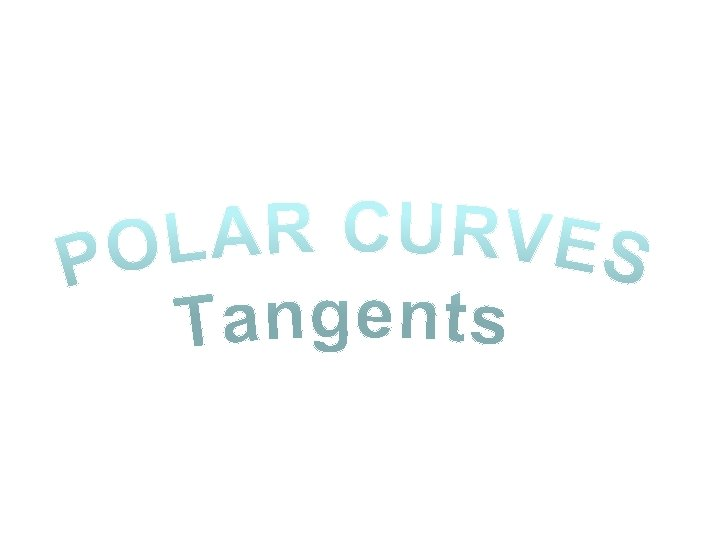Polar curves KUS objectives BAT Find Tangents to Polar curves Starter: Sketch these graphs:Notes To start with we can look at using the Polar equation to find tangents to a curve that are parallel or perpendicular to the original line Differentiating and setting the expression equal to 0 The line from the origin at an angle of 0 is called the ‘initial line’ The equation y = rsinθ represents changes in the vertical direction When dy/dθ is 0, that means that there is no movement in the vertical direction (the change in y with respect to a change in θ is 0) Therefore, if dy/ dθ is 0, the curve is parallel to the ‘initial line’Notes The line from the origin at an angle of 0 is called the ‘initial line’ The equation x = rcosθ represents changes in the horizontal direction When dx/dθ is 0, that means that there is no movement in the horizontal direction (the change in x with respect to a change in θ is 0) Therefore, if dy/ dθ is 0, the curve is perpendicular to the ‘initial line’WB 22 Find the coordinates of the points on: r = a(1 + cosθ) Where the tangents are parallel to the initial line θ = 0 Find θ in the range 0 ≤ θ < 2π (3 a/2, π/3) Find θ in the range 0 ≤ θ < 2π Use these to find r so you have the full coordinates So the curve is parallel to the initial line in these positions: (0, π) (3 a/2, -π/3)WB 23 a Find the coordinates and the equations of the tangents to the curve : r = asin 2θ, 0 ≤ θ ≤ π/2 Where the tangents are: a) Parallel to the initial line Give answers to 3 s. f where appropriate: (2 a√ 2/3, 0. 955) Sketch it to get an idea of where the tangents will be… The equation of this line is just: (0, 0) If dy/dθ = 0, then the part in the bracket must be 0 Replace sin 2θ and cos 2θ with equivalent expressions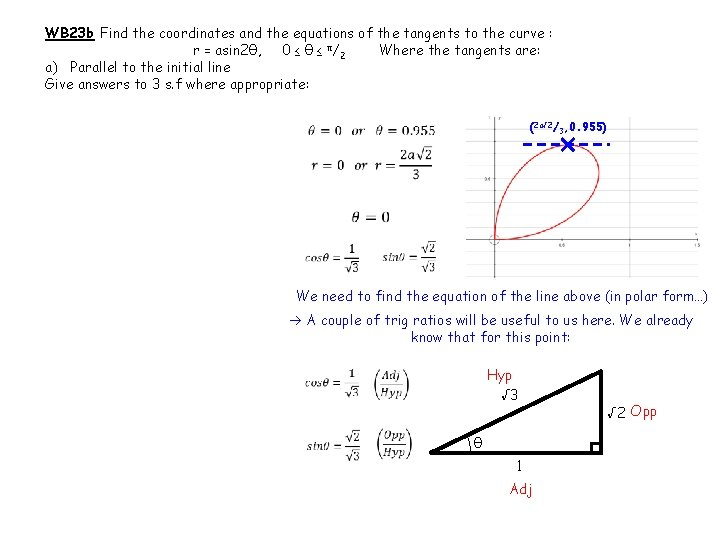WB 23 b Find the coordinates and the equations of the tangents to the curve : r = asin 2θ, 0 ≤ θ ≤ π/2 Where the tangents are: a) Parallel to the initial line Give answers to 3 s. f where appropriate: (2 a√ 2/3, 0. 955) We need to find the equation of the line above (in polar form…) A couple of trig ratios will be useful to us here. We already know that for this point: Hyp √ 3 θ 1 Adj √ 2 Opp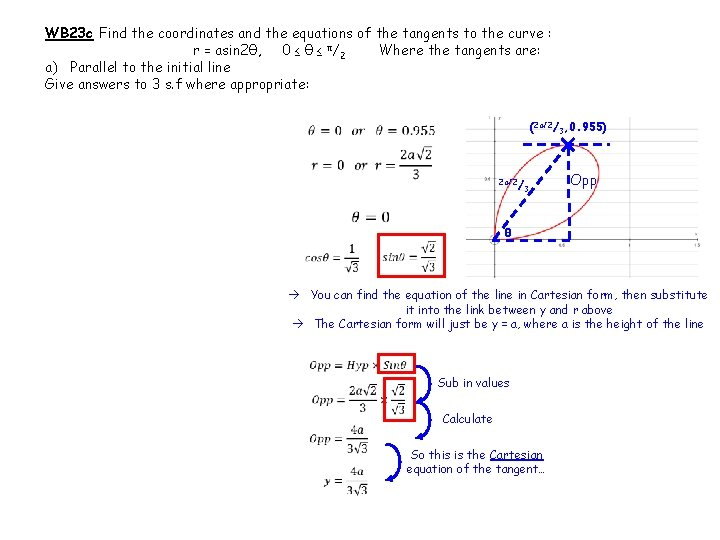WB 23 c Find the coordinates and the equations of the tangents to the curve : r = asin 2θ, 0 ≤ θ ≤ π/2 Where the tangents are: a) Parallel to the initial line Give answers to 3 s. f where appropriate: (2 a√ 2/3, 0. 955) 2 a√ 2/ 3 θ Opp You can find the equation of the line in Cartesian form, then substitute it into the link between y and r above The Cartesian form will just be y = a, where a is the height of the line Sub in values Calculate So this is the Cartesian equation of the tangent…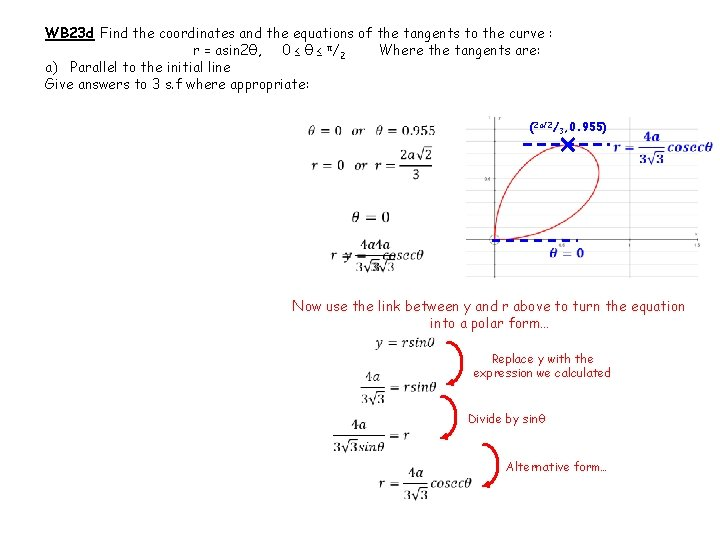WB 23 d Find the coordinates and the equations of the tangents to the curve : r = asin 2θ, 0 ≤ θ ≤ π/2 Where the tangents are: a) Parallel to the initial line Give answers to 3 s. f where appropriate: (2 a√ 2/3, 0. 955) Now use the link between y and r above to turn the equation into a polar form… Replace y with the expression we calculated Divide by sinθ Alternative form…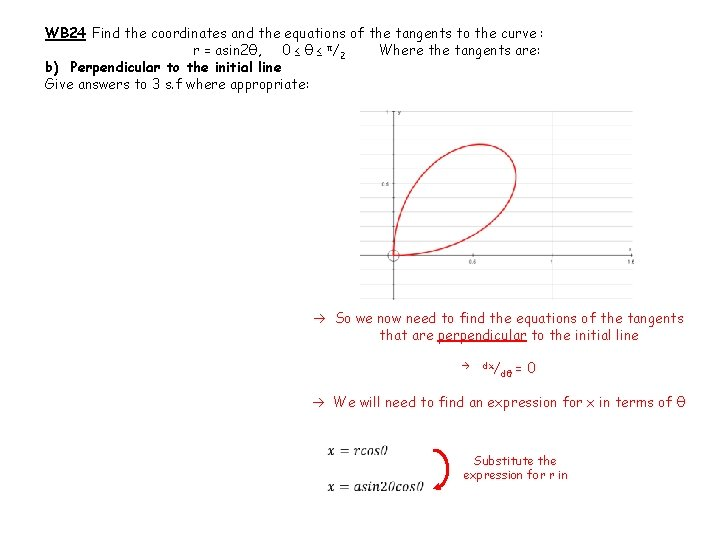WB 24 Find the coordinates and the equations of the tangents to the curve : r = asin 2θ, 0 ≤ θ ≤ π/2 Where the tangents are: b) Perpendicular to the initial line Give answers to 3 s. f where appropriate: So we now need to find the equations of the tangents that are perpendicular to the initial line dx/ dθ = 0 We will need to find an expression for x in terms of θ Substitute the expression for r in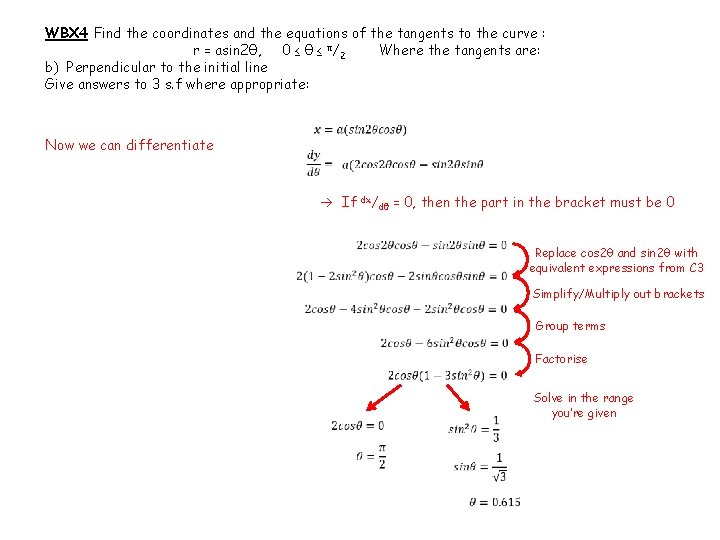WBX 4 Find the coordinates and the equations of the tangents to the curve : r = asin 2θ, 0 ≤ θ ≤ π/2 Where the tangents are: b) Perpendicular to the initial line Give answers to 3 s. f where appropriate: Now we can differentiate If dx/dθ = 0, then the part in the bracket must be 0 Replace cos 2θ and sin 2θ with equivalent expressions from C 3 Simplify/Multiply out brackets Group terms Factorise Solve in the range you’re givenWBX 4 Find the coordinates and the equations of the tangents to the curve : r = asin 2θ, 0 ≤ θ ≤ π/2 Where the tangents are: b) Perpendicular to the initial line Give answers to 3 s. f where appropriate: (2 a√ 2/3, 0. 615) (0, π/2) The equation of this line is just: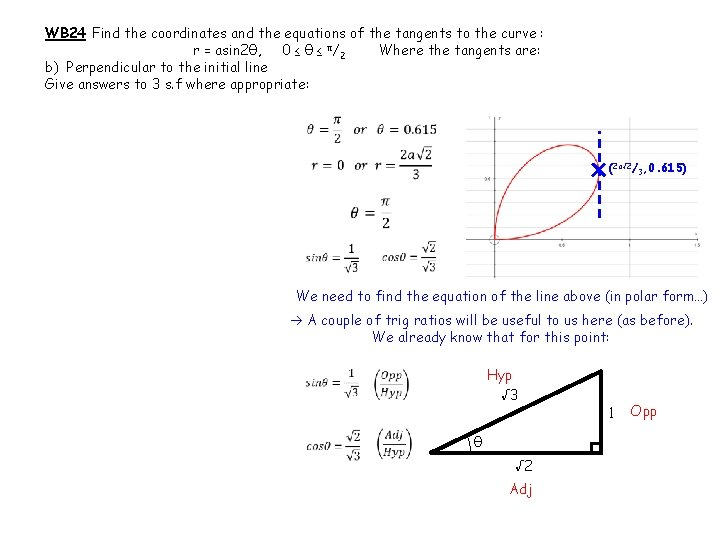WB 24 Find the coordinates and the equations of the tangents to the curve : r = asin 2θ, 0 ≤ θ ≤ π/2 Where the tangents are: b) Perpendicular to the initial line Give answers to 3 s. f where appropriate: (2 a√ 2/3, 0. 615) We need to find the equation of the line above (in polar form…) A couple of trig ratios will be useful to us here (as before). We already know that for this point: Hyp √ 3 θ √ 2 Adj 1 OppWB 24 Find the coordinates and the equations of the tangents to the curve : r = asin 2θ, 0 ≤ θ ≤ π/2 Where the tangents are: b) Perpendicular to the initial line Give answers to 3 s. f where appropriate: 2 a√ 2/ 3 (2 a√ 2/3, 0. 615) θ Adj You can find the equation of the line in Cartesian form, then substitute it into the link between y and r above The Cartesian form will just be x = a, where a is the horizontal distance of the line from the origin Sub in values Calculate So this is the Cartesian equation of the tangent…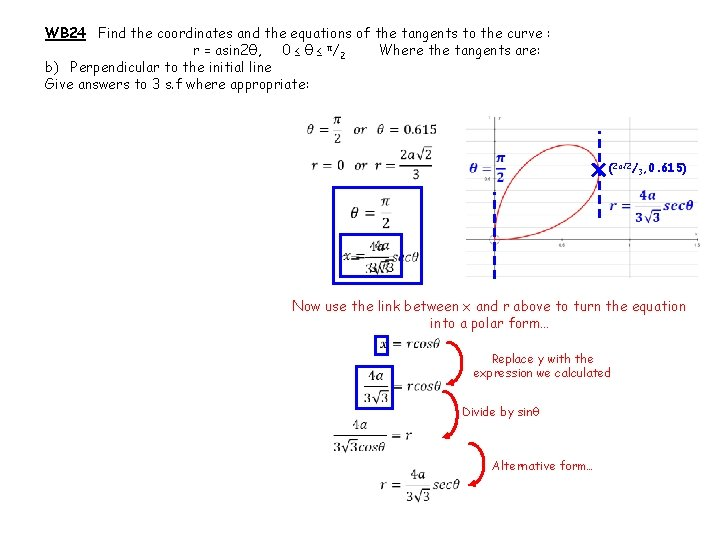WB 24 Find the coordinates and the equations of the tangents to the curve : r = asin 2θ, 0 ≤ θ ≤ π/2 Where the tangents are: b) Perpendicular to the initial line Give answers to 3 s. f where appropriate: (2 a√ 2/3, 0. 615) Now use the link between x and r above to turn the equation into a polar form… Replace y with the expression we calculated Divide by sinθ Alternative form…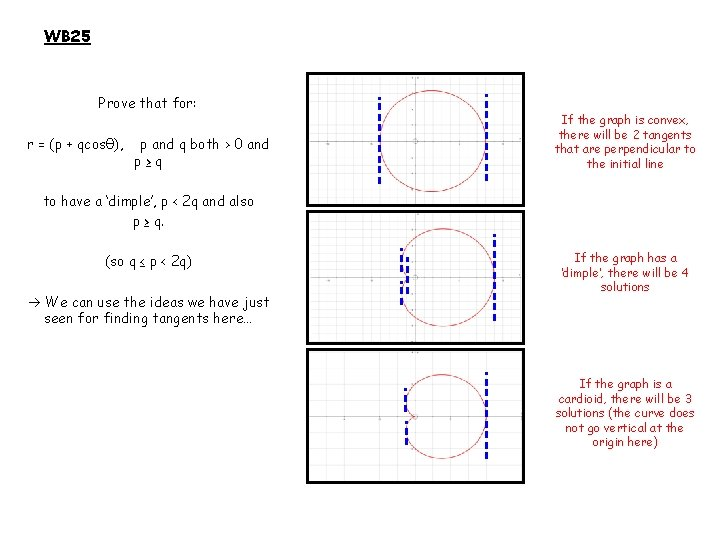WB 25 Prove that for: r = (p + qcosθ), p and q both > 0 and p≥q If the graph is convex, there will be 2 tangents that are perpendicular to the initial line to have a ‘dimple’, p < 2 q and also p ≥ q. (so q ≤ p < 2 q) We can use the ideas we have just seen for finding tangents here… If the graph has a ‘dimple’, there will be 4 solutions If the graph is a cardioid, there will be 3 solutions (the curve does not go vertical at the origin here)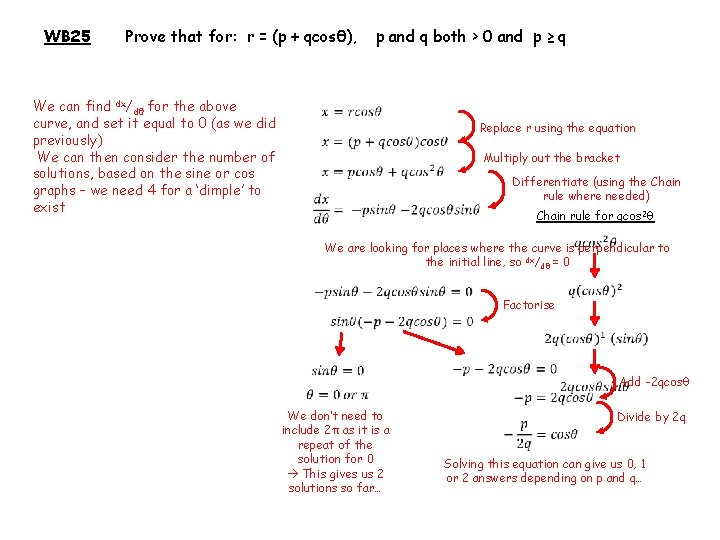WB 25 Prove that for: r = (p + qcosθ), We can find dx/dθ for the above curve, and set it equal to 0 (as we did previously) We can then consider the number of solutions, based on the sine or cos graphs – we need 4 for a ‘dimple’ to exist p and q both > 0 and p ≥ q Replace r using the equation Multiply out the bracket Differentiate (using the Chain rule where needed) Chain rule for qcos 2θ We are looking for places where the curve is perpendicular to the initial line, so dx/dθ = 0 Factorise We don’t need to include 2π as it is a repeat of the solution for 0 This gives us 2 solutions so far… Add -2 qcosθ Divide by 2 q Solving this equation can give us 0, 1 or 2 answers depending on p and q…WB 25 Prove that for: r = (p + qcosθ), -1 Cosθ 1 0 p and q both > 0 and p ≥ q π/ 2 π 3π/ 2 2π As the value for cosθ is negative, it must be between π/2 and 3π/2 If p > 2 q If p < 2 q Eg) p = 5, q = 1 Eg) p = 3, q = 2 The fraction will be top-heavy (in this case -5/2) The fraction will be ‘regular’ (in this case -3/4) Cosθ will be less than -1 Cosθ will be between 0 and -1 No solutions in this range 2 solutions in this range If p = 2 q So p ≥ q Eg) p = 6, q = 3 and Cos θ = -1 1 solution (θ = π) p < 2 q Therefore: q ≤ p <2 q If p was not greater than q, there would be a lot of undefined areas on the graph, and hence the full shape would not exist (there may actually be no defined areas at all)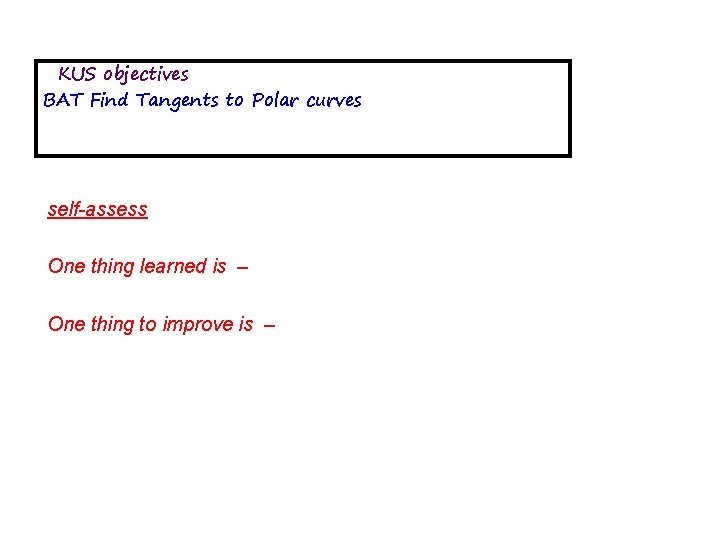KUS objectives BAT Find Tangents to Polar curves self-assess One thing learned is – One thing to improve is –END